## Sunday, June 24, 2018

### Task 2 - Drawing Similar Triangles (Part 2)

Task 2: Drawing Similar Triangles

In the applet below, A'B' = 2AB, A'C'= 2AC, and B'C'= 2BC. Use the Segment tool to draw ∆PQR such that PQ = 3AB, PR= 3AC, and QR = 3BC.

Questions

1.) How did you draw ∆PQR?

2.) What do you observe about the corresponding angles of the ∆ABC, ∆A'B'C' and ∆PQR?

3.) Based on Task 1 and Task 2, make a conjecture about your observations above.

Discussion

In Task 1 and Task 2, you have observed that it is easier to draw similar triangles if you make the corresponding sides of the triangles parallel. In addition, you have also observed that the corresponding angles of the triangles are congruent. For example,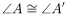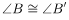and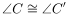.

In drawing the triangles, you made sure that the ratio of the lengths of corresponding sides is equal. In other words, you made the length of the corresponding sides proportional. For instance, the ratio of the lengths of the corresponding sides of to those of is 2. That is,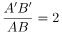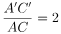and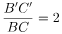Two triangles whose corresponding angles are congruent are called similar triangles. The lengths of the corresponding sides of similar triangles are proportional.

1.The exercises are very interesting, thank for sharing with us
fanfic :best story

2.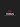3.Download the official Showbox app here to stream or download free movies and TV shows on your Android device to Watch Showbox Movies Online Free download file and step by step tutorial.

4.5.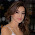Are you gonna write an essay? You'd better order an editing service for it. have a look here to find it!

6.That's nice tip for me. I have a presentation next week windows 10

7.